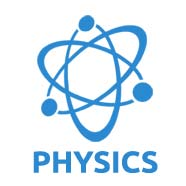## Pages

### Physics GK In Hindi

Physics GK Question
Quiz 2

Que: If a wire of resistance R is melted and recast to half of its length, then the new resistance of the wire will be
(A) R/4
(B) R/2
(C) R
(D) 2R

Que: A non-electronic conductor is
(A) Iron
(B) Gas Carbon
(C) Copper Sulphate
(D) None

Que: Ohm’s law is valid in case of
(A) Semiconductor
(B) Conductor
(C) Superconductor
(D) Insulator

Que : The Laser is a beam of radiations which are
(A) Non-coherent and monochromatic
(B) Coherent and monochromatic
(C) Non-coherent and non-monochromatic
(D) Coherent and non-monochromatic

Que: Centigrade and Fahrenheit temperatures are the same at
(A) 320
(B) 400
(C) -2730
(D) -400

Que: The dimensional formula for universal gravitational constant is
(A) M-1L3T2
(B) ML2T-2
(C) M-2
(D) M-1L3T-2

Que: Diopter is the unit of
(A) Power of a lens
(B) Focal length of a lens
(C) Light intensity
(D) Sound intensity
Answer: (A) Power of a lens

Que : When a atone is thrown in a calm water of a pond the waves produced on the surface of water are
(A) Longitudinal
(B) Transverse
(C) Both A and B
(D) Waves are not produced

Que: In a filament type light bulb most of the electric power consumped appears as
(A) Visible light
(B) Infra red light
(C) Ultra violet rays
(D) Fluorescent light

Que: The non metal found in the liquid state is
(A) Bromine
(B) Nitrogen
(C) Fluorine
(D) Chlorine